# 让训练更长序列模型称为可能Sequence Parallelism_zzk

## Sequence Parallelism¶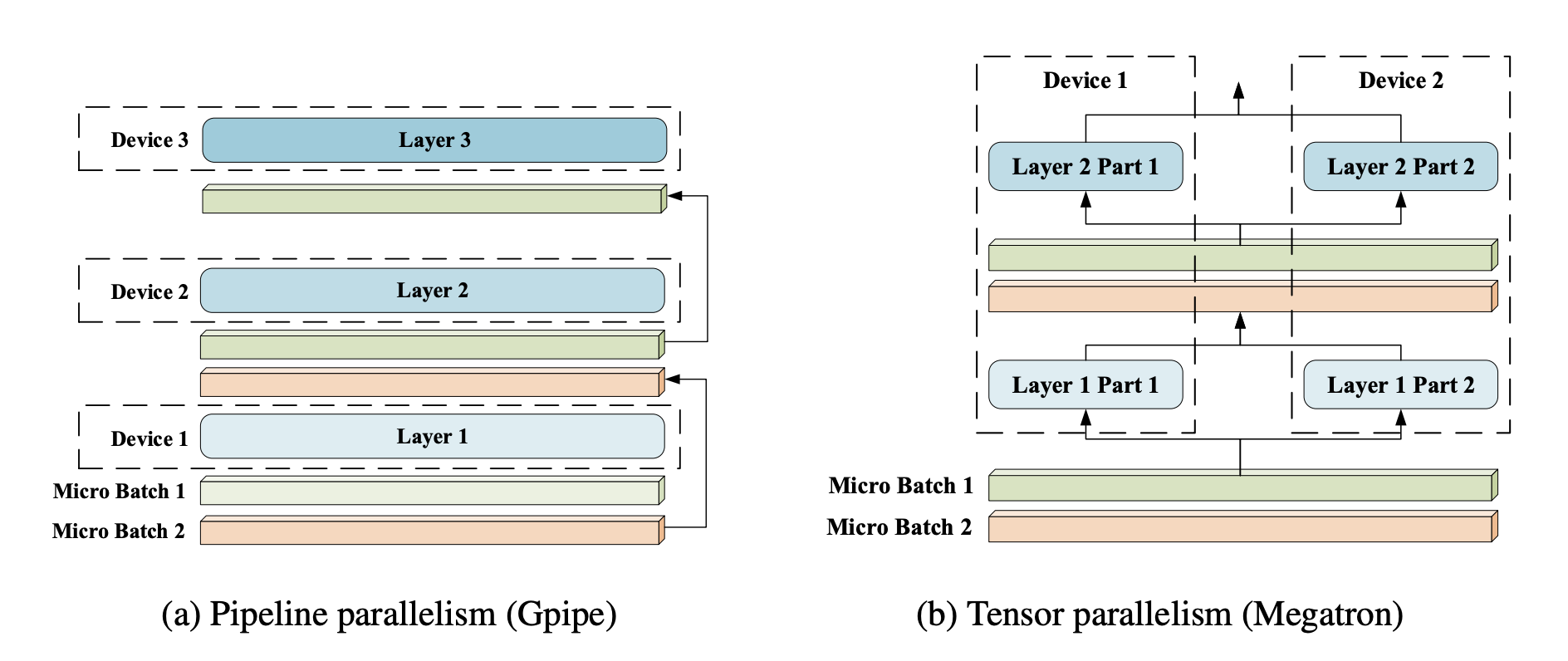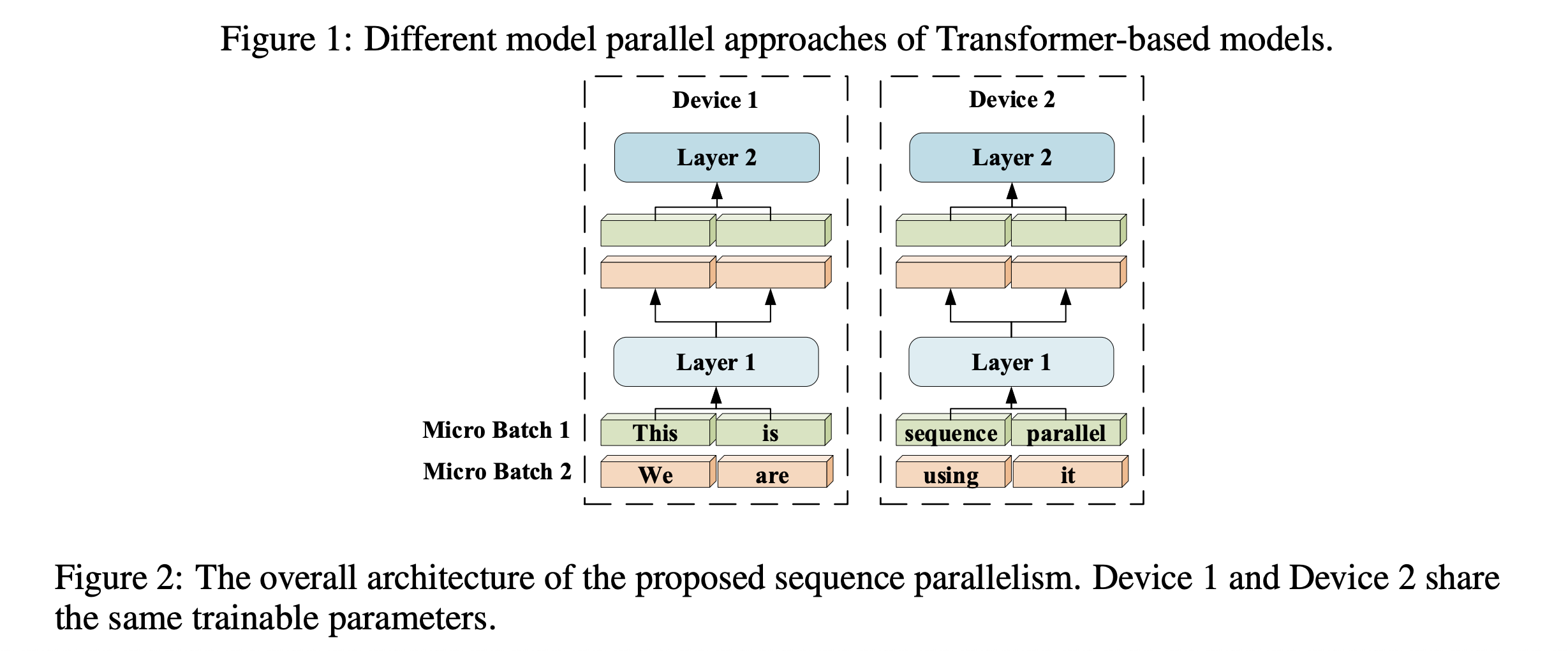• B batch size
• L 序列长度
• H 全连接层维度大小
• A 注意力头维度大小
• Z 注意力头的个数
• N GPU个数

## MLP部分的序列并行¶

// 假设输入是 (B, L, H)
dense1 = nn.Linear(H, 4H)
dense2 = nn.Linear(4H, H)


\frac{4H^2}{N}*2(两个全连接层参数大小)
H*\frac{4H^2}{N}*2(两个全连接层参数对应梯度)
(第一层全连接输出结果激活值) \\ \frac{4BLH}{N}
(第二层全连接输出结果激活值) \\ BLH

\frac{32H^2}{N} + \frac{4BLH}{N} + BLH

4H*H*4 + \frac{4BLH}{N} + \frac{BLH}{N}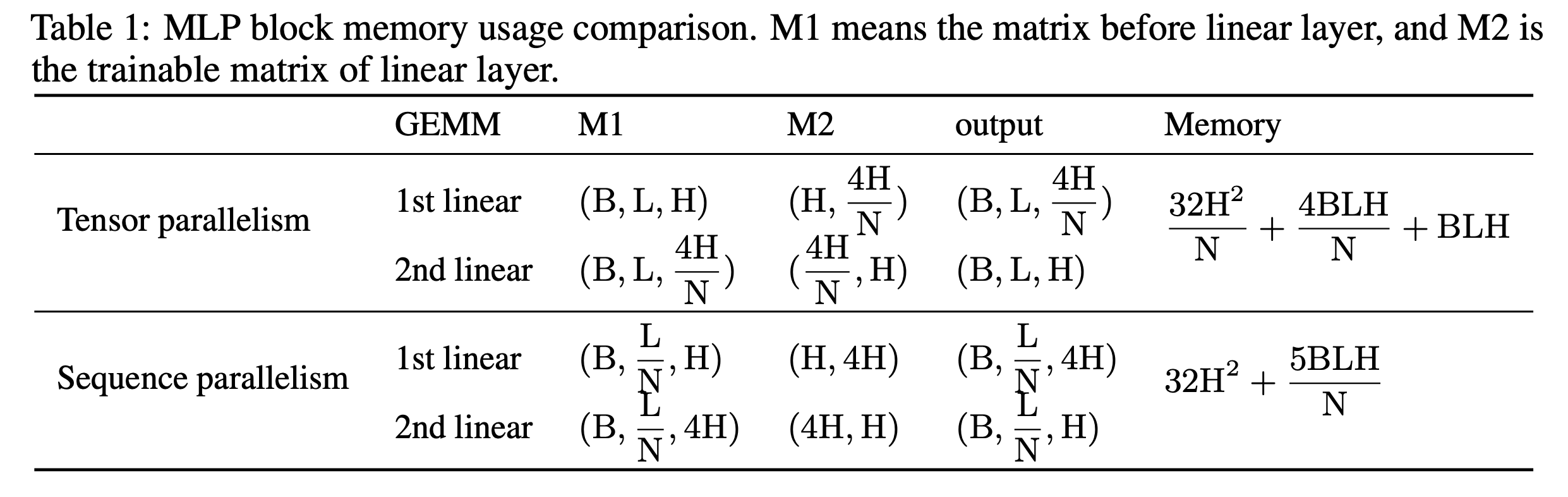## 自注意力机制的序列并行¶

### 补充材料：Ring Allreduce¶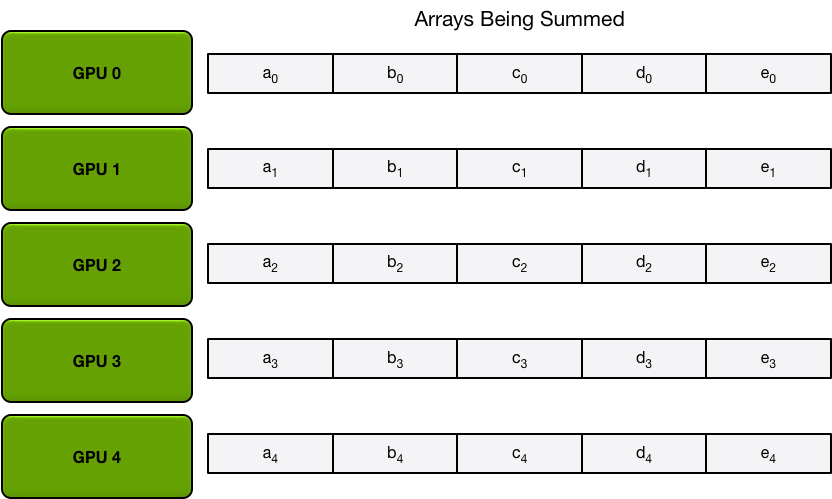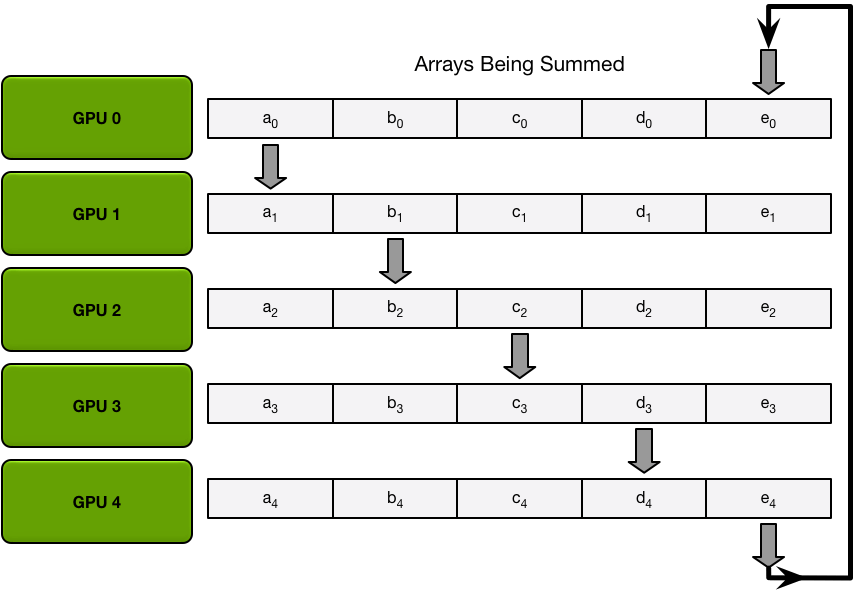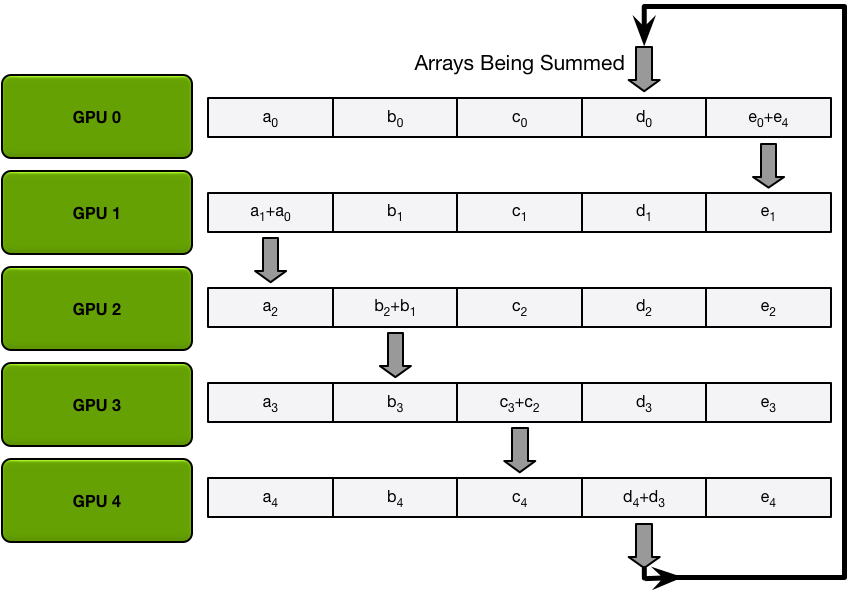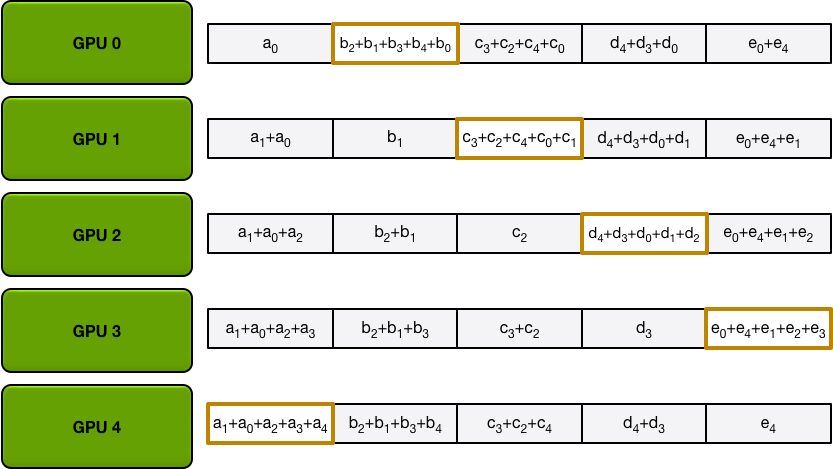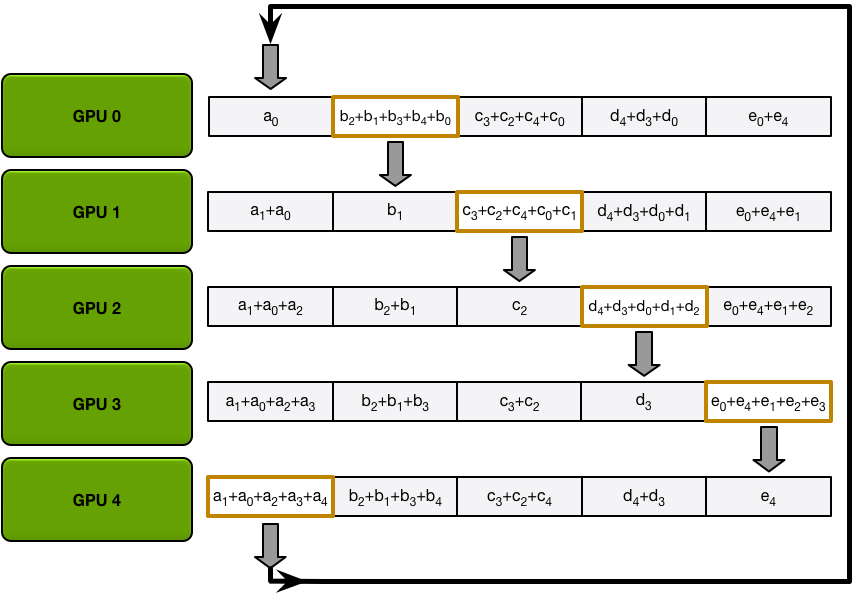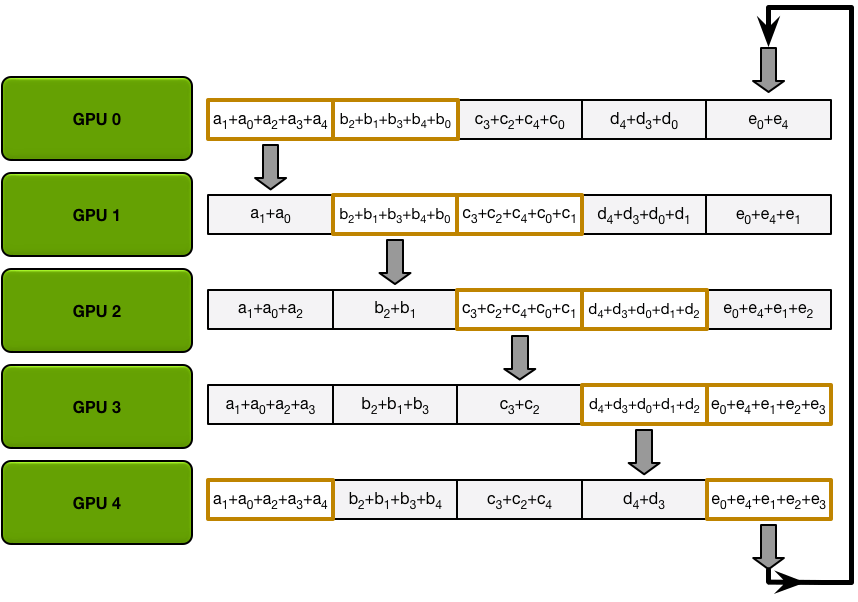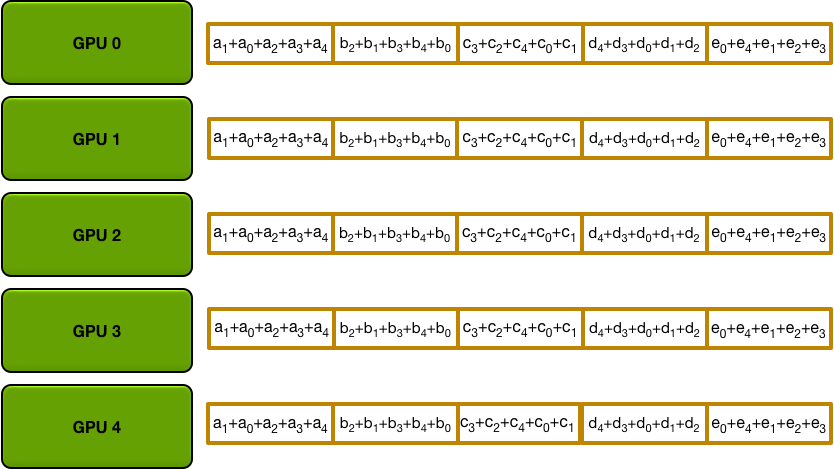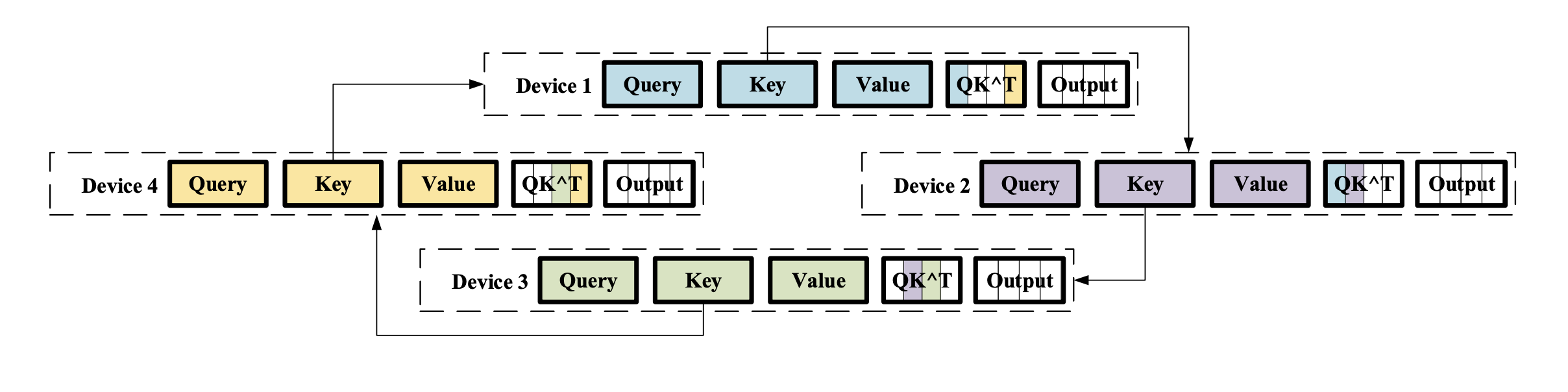• Device1接收了Device4上的Key，计算了Device1，Device4的Q1K
• Device2接收了Device1上的Key，计算了Device2，Device1的Q2K
• Device3接收了Device2上的Key，计算了Device3，Device2的Q3K
• Device4接收了Device3上的Key，计算了Device4，Device3的Q4K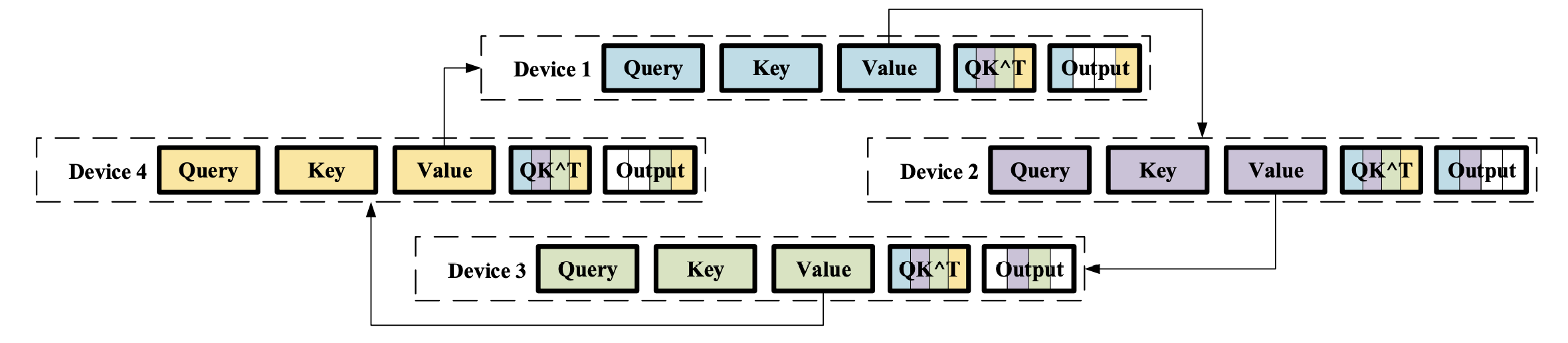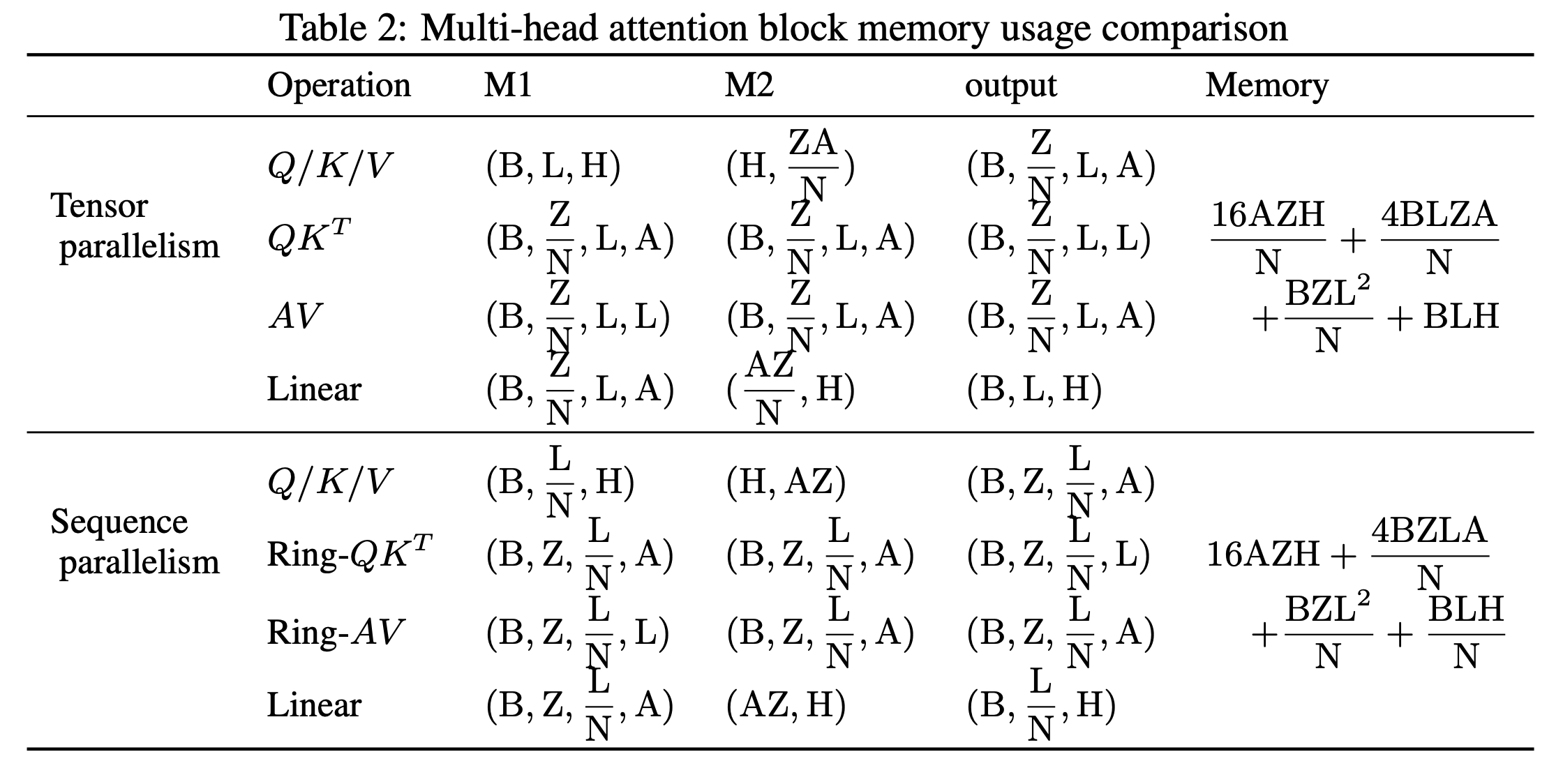## 实验结果¶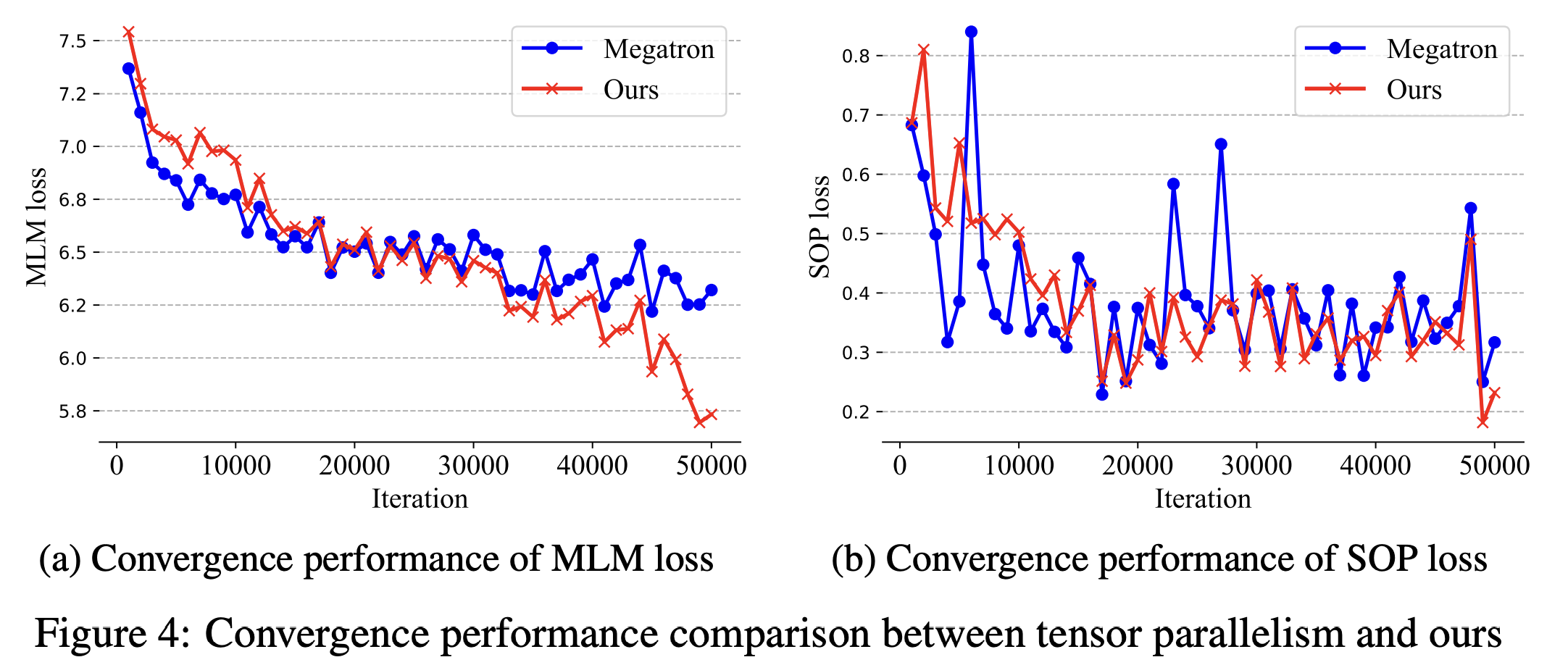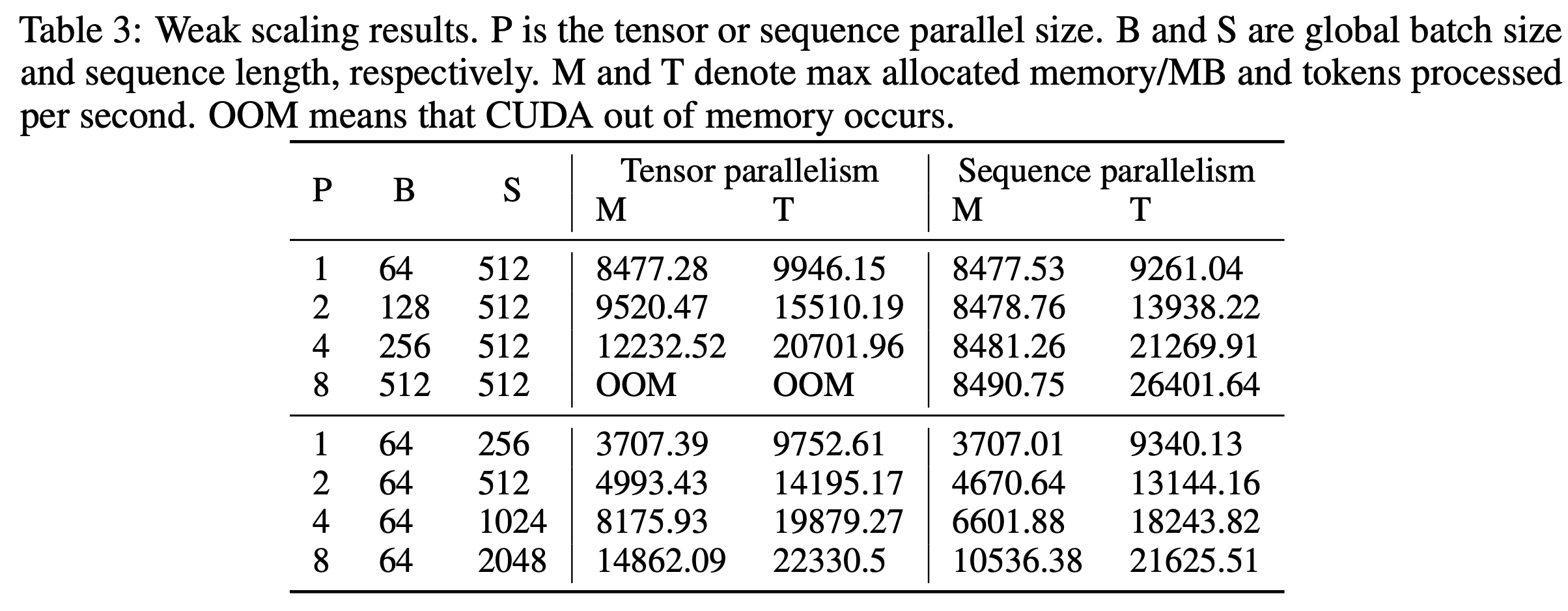## 代码简单走读¶

colossalai/nn/layer/parallel_sequence/layers.py 这里有对应的序列并行的API TransformerSelfAttentionRing

class RingQK(torch.autograd.Function):
@staticmethod
def forwardctx,
sub_q,
sub_k,
batch_size,
sub_seq_length):
# 首先创建一个存放attention_score的空间
attention_score = torch.empty(
...
)
# 根据当前设备的QK，计算local QK结果
part_a = torch.matmul(sub_q, sub_k.transpose(2, 1))
local_rank = gpc.get_local_rank(ParallelMode.SEQUENCE)
local_world_size = gpc.get_world_size(ParallelMode.SEQUENCE)
start_idx = local_rank * sub_seq_length
end_idx = (local_rank + 1) * sub_seq_length
# 使用切片，将local QK结果存放到attention_score
attention_score[:, :, start_idx: end_idx] = part_a

for i in range(local_world_size - 1):
# 调用ring_forward函数，传输本卡上的Key
# 接收相邻卡的Key
sub_k = ring_forward(sub_k, ParallelMode.SEQUENCE)
start_idx, end_idx = _calc_incoming_device_range(i, local_rank, local_world_size, sub_seq_length)
# 使用当前的Q，和相邻卡的Key计算Attention score
part_a = torch.matmul(sub_q, sub_k.transpose(2, 1))
# 存放结果
attention_score[:, :, start_idx:end_idx] = part_a
# 如此循环至Q与各个device的Key都运算过，得到完整的Attention Scores.
return attention_score


# send to next rank
send_next_op = torch.distributed.P2POp(
torch.distributed.isend, tensor_send_next,
gpc.get_next_global_rank(parallel_mode))
ops.append(send_next_op)

# receive from prev rank
recv_prev_op = torch.distributed.P2POp(
torch.distributed.irecv, tensor_recv_prev,
gpc.get_prev_global_rank(parallel_mode))
ops.append(recv_prev_op)